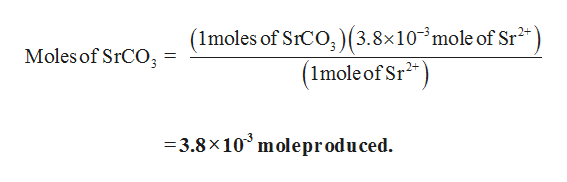# An aqueous solution containing both Sr2+ and CO32– ions is mixed in order to precipitate SrCO3(s). Given there are 1.50 mL of 2.53 MSr2+ ions, calculate the mass of SrCO3 that is formed. Assume that all of the Sr2+ ions are consumed. The net ionic reaction is shown below.Sr^2+(aq)+CO2^−3​(aq) --> SrCO3​(s)____ g

Question
69 views

An aqueous solution containing both Sr2+ and CO32– ions is mixed in order to precipitate SrCO3(s). Given there are 1.50 mL of 2.53 MSr2+ ions, calculate the mass of SrCO3 that is formed. Assume that all of the Sr2+ ions are consumed. The net ionic reaction is shown below.

Sr^2+(aq)+CO2^−3​(aq) --> SrCO3​(s)

____ g

check_circle

Step 1

The given balanced reaction is,

Step 2

Hence, 1 mole of Sr2+ produces 1 mole of SrCO3, then

3.8x10-3 moles of Sr2+produces ‘x’ moles of SrCO3

The moles of SrCO3 is calculated as shown,

...help_outlineImage Transcriptionclose(1moles of SICO, )(3.8x103mole of Sr2 Moles of SrCO3 (Imole of Sr =3.8x 10 moleprodu ced. fullscreen

### Want to see the full answer?

See Solution

#### Want to see this answer and more?

Solutions are written by subject experts who are available 24/7. Questions are typically answered within 1 hour.*

See Solution
*Response times may vary by subject and question.
Tagged in

### General Chemistry